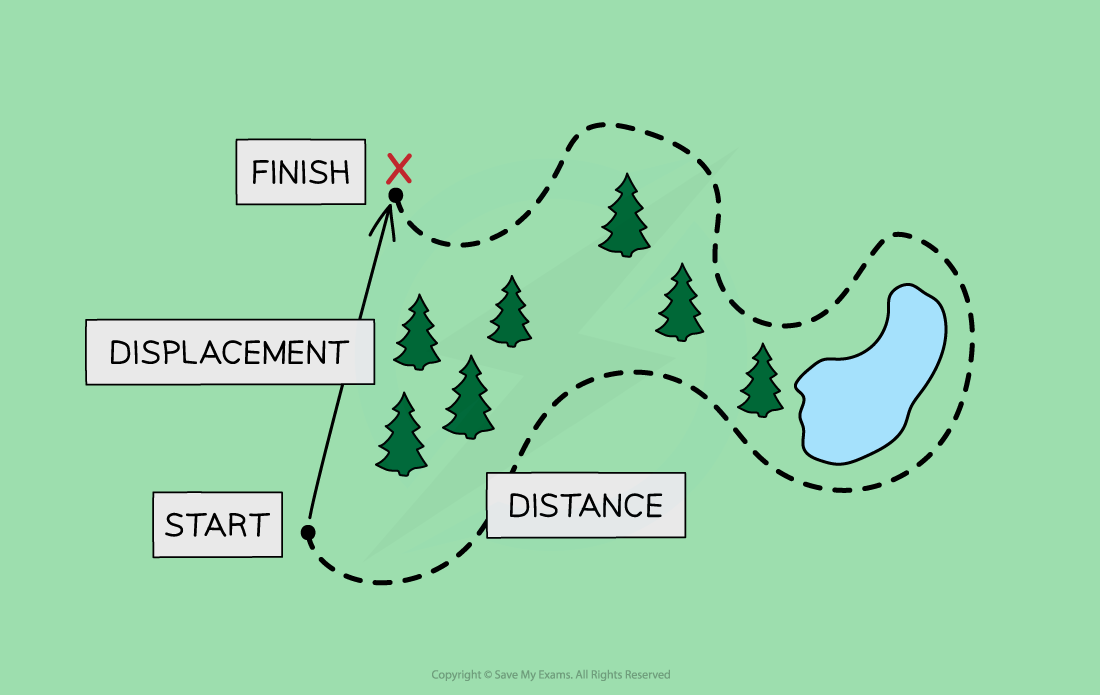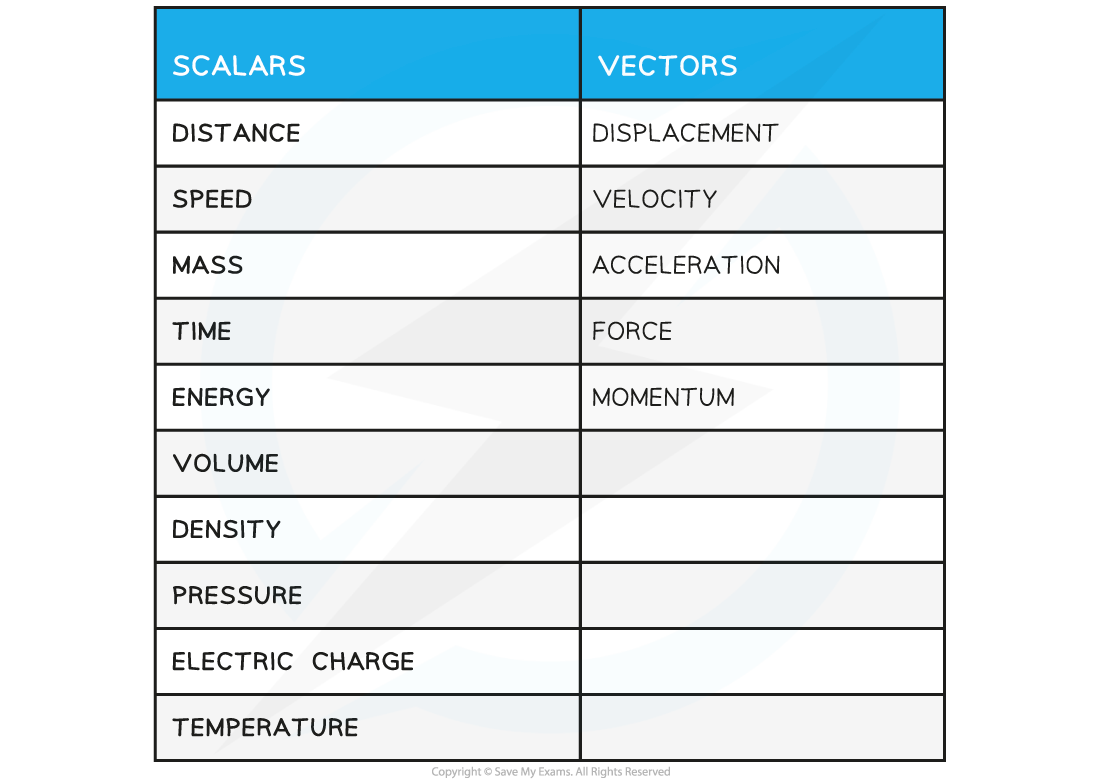# IB DP Physics: SL复习笔记1.3.1 Vector & Scalar Quantities

### Vector & Scalar Quantities

• A scalar is a quantity which only has a magnitude (size)
• A vector is a quantity which has both a magnitude and a direction
• For example, if a person goes on a hike in the woods to a location which is a couple of miles from their starting point
• As the crow flies, their displacement will only be a few miles but the distance they walked will be much longerDisplacement is a vector while distance is a scalar quantity

• Distance is a scalar quantity
• This is because it describes how an object has travelled overall, but not the direction it has travelled in
• Displacement is a vector quantity
• This is because it describes how far an object is from where it started and in what direction
• Some common scalar and vector quantities are shown in the table below:

Scalars and Vectors Table#### Exam Tip

Do you have trouble figuring out if a quantity is a vector or a scalar? Just think - can this quantity have a minus sign? For example - can you have negative energy? No. Can you have negative displacement? Yes!